Information About Quadratic Graphs MathBitsNotebook.com Terms of Use   Contact Person: Donna Roberts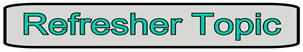The graphs of quadratic functions,  f (x) = ax2 + bx + c, are called parabolas.Shape of the Graph: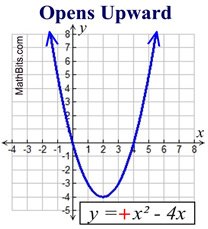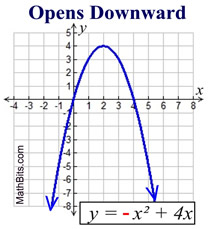Parabolas have a shape that resembles (but is not the same as) the letter U. Parabolas may open upward or downward. If the sign of the leading coefficient, a, is positive (a > 0), the parabola opens upward. If the sign of the leading coefficient, a, is negative (a < 0), the parabola opens downward.Parts of the Graph: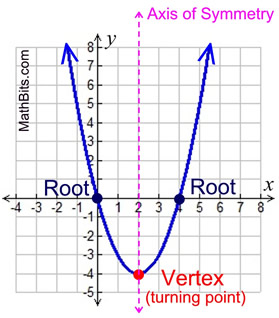The bottom (or top) of the U is called the vertex, or the turning point. The vertex of a parabola opening upward is also called the minimum point. The vertex of a parabola opening downward is also called the maximum point. The x-intercepts are called the roots, or the zeros. To find the x-intercepts, set ax2 + bx + c = 0. The ends of the graph continue to positive infinity (or negative infinity) unless the domain (the x's to be graphed) is otherwise specified.Axis of Symmetry:
The parabola is symmetric (a mirror image) about a vertical line drawn through its vertex (turning point). This line is called the axis of symmetry. The equation for the axis of symmetry is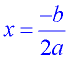.
 Parabola:   y = x2 + 4x - 5 Axis of symmetry: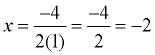x = -2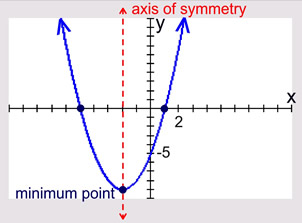Parabola:   y = -x2 + x + 6 Axis of symmetry: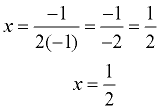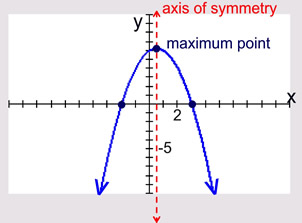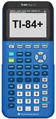For calculator help with graphing parabolas click here.